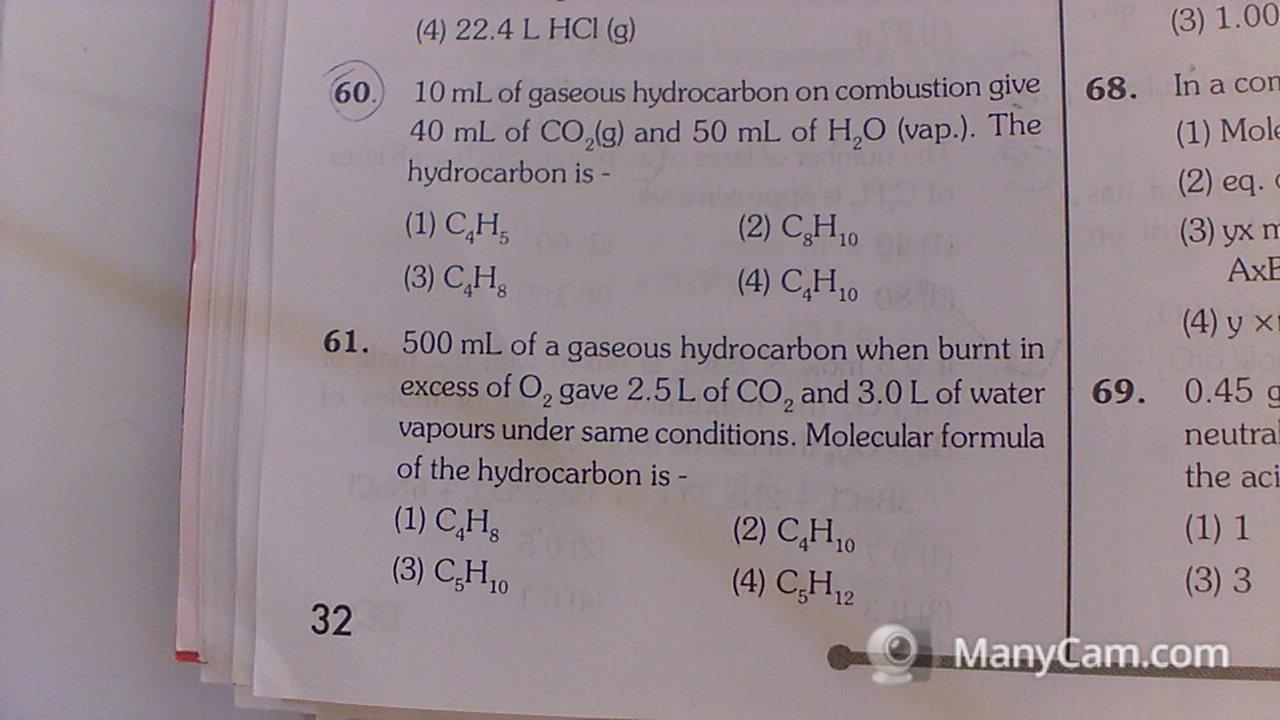#### My_Snapshot_11.jpg10 mL of gaseous hydrocarbon on combustion give 40 mL of C02(g) and 50 mL of H20 (vap.). The hydrocarbon is -

Question 60-

first solution is -

10 mL of gaseous hydrocarbon on combustion gives 40 mL of CO2

The number of C atoms in the formula unit is 40/10×1=4

and
10 mL of gaseous hydrocarbon on combustion gives 50 mL of H2O
50/10×2 =10
The hydrocarbon is C4H10

Second solution is:-

the ratio of volumes is
10 : 40 : 50
1 : 4 : 5

all the Carbon from the hydrocarbon is in the CO2
10 ml of hydrocarbon produces 40 ml CO2,
1 : 4 ratio
So 1 mole hydrocarbon has 4 moles Carbon

All the H ends up in the water
10 ml hydrocarbon produces 50 ml water,
1 : 5 ratio,
but also each water has 2 H atoms
So 1 mole hydrocarbon has 10 moles Hydrogen

which gives
C4H10

You can balance an equation for combustion of C4H10 to check the answer

C4H10 + 13/2 O2 --> 4CO2 + 5H2O

#### Ankit